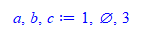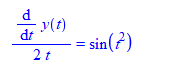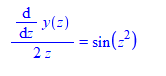## ODE plot not working...

Don't get a DEplot

 > with(DEtools,DEplot);with(plots,display);(1)
 >
 >
 >
 > init3:=seq([0,k*0.2,0],k=-4..10);(2)

The forward plot is p3:

 > p3:=DEplot([sys],[x,y],t=0..2,[init3],x=-3..2,y=-3..2,stepsize=.05,arrows=none,linecolor=black,thickness=1,axes=box,scaling=constrained):

he backward plot is np3:

 > np3:=DEplot([sys],[x,y],t=-2..0,[init3],x=-3..2,y=-3..2,stepsize=.05,arrows=none,linecolor=black,thickness=1,axes=box,scaling=constrained):
 Warning, plot may be incomplete, the following errors(s) were issued:    cannot evaluate the solution further left of -.29861232, probably a singularity Warning, plot may be incomplete, the following errors(s) were issued:    cannot evaluate the solution further left of -.93147185e-1, probably a singularity Warning, plot may be incomplete, the following errors(s) were issued:    cannot evaluate the solution further left of -.23648931e-1, probably a singularity Warning, plot may be incomplete, the following errors(s) were issued:    cannot evaluate the solution further left of -.27325542e-2, probably a singularity
 >
 > display({p1,p2,p3,np3});
 >

## on returning multiple results from a proc...

Maple can return multiple values as expression sequence. The problem with this is that if one of the values is NULL, then one gets an error on the receiving end.

For example, suppose a proc foo() is meant to return 3 separate values. When doing

a,b,c := foo()

This will fail if one of the values was NULL. For example, if b  was NULL, then Maple returns a,c only. The NULL value is discarded.

```foo:=proc()
local a,b,c;
a:=1; b:=NULL; c:=3;
return a,b,c;
end proc;

a,b,c := foo()

```

Error, mismatched multiple assignment of 3 variables on the left side and 2 values on the right side

I'd like to use NULL to indicate that this variable has no value (may be it could be something that could not be computed inside foo, or some solution that could not be found, and for any other reason.)

So currently I use {} instead of NULL everywhere to indicate no value for that variable and that works

```foo:=proc()
local a,b,c;
a:=1; b:={}; c:=3;
return a,b,c;
end proc;

a,b,c := foo()
```And now I check using if b={} instead of if b=NULL and that works OK for what I want.

It would be nice if one could do this

[a,b,c] := foo()

And even if b was NULL, it will still keep that slot. But Maple will also replace [a,b,c]  with [a,c]  if b was NULL

So what is NULL used for in Maple exactly? (help says it is used to initialize an expression sequence). Since one can't return NULL as value.

In Mathematica, NULL can be returned as an actual value. For example

```foo[] := Module[{a, b, c},
a = 1; b = Null; c = 3;
{a, b, c}
];

{a, b, c} = foo[]

```

gives

{1, Null, 3}

My question: is using {} in place of NULL a common practice  in Maple to indicate no value for a variable being returned? Or is there a better way than this?

## Maple 2020 & MacOS 11...

I have been using Maple 2017 but recently upgraded to MacOS Catalina. It doesn't work anymore. I would upgrade to Maple 2020, but worried will I have to upgrade again when the new MacOS 11 comes out? does anyone have any experiece dealing with similar situation on previous MacOS upgrade?

## How to get rid of 1.0 in numerical and symbolic ca...

Hi,

This might be a very silly question but it is troubling me a little bit and that's why I need to post it. During the combination of symbolic and numerical comutation this '1.0' is appearing as a coefficient for the variables whose coeffecient is just '1'. It's quite annoying as sometimes if I have to collect coefficient of a variable for an example exp(I*omega*t) then I have to write exp(1.I*omega*t), so chances of making mistake is higher. Please find the attached worksheet for this. In eq(4), you can easily see that for x(t) and x(t) this '1.0' appears in front of the variables.

I really appreciate  if someone can help me out of this.

With Regards

Sunitquestion.mw

 >>>>>>>>>>>>>>>(1)
 >(2)
 >(3)
 >(4)
 >## What is the conception of “process” and “task” key...

Dear All,

I am studying parallel programming in Maple. Two words are important keys in parallel programming. The one is “task”, and another is “process”. In the help pages of Maple are said the Grid:-Map package is executed in separate “processes”, while the Threads:-Map command is tried to divide the input into separate “tasks”.

1. What is the difference between the “process” and “task” conception and meaning in Threads and Grid packages?
2. What is the discrepancy between Grid:-Map and Threads:-Map commands in the practice and execution?
3. Between the “process” and “task”, which one of them does have the smaller run-time?
4. What is the default value of “tasksize” option in Grid:-Map and Threads:-Map commands,
5. Does or the larger value of the “tasksize” option results in smaller run-time either the smaller value of that?

Can anyone explain to me the above questions?

Best wishes

## how to determine unique symbol to use inside a pro...

What is a good way to determine, at run time, a free symbol to use inside a proce, so it does not appear the same as one being passed in from outside?

Here is the problem. Inside a proc, user calls it with an ODE. The independent variable is passed in as the argument called x

Inside this proc, it does change of variables to do something else.  If the proc uses say as the new variabe, this could be the same as the passed in argument in the way it appears when being printed out to file.

I know the local symbol is not the same as the passed in symbol, even if that happend to be also. As one is local and the other is not. So the math will still work, but the display will look very confusing to the user.

But since I am printing these out, these will show the same.

So I need a way to make symbol inside proc, which looks different from the one being passed in.

An example will show the problem.

```foo:=proc(ode::`=`,y,x)
local t; #hoping this will not be the same looking as actual x
return PDEtools:-dchange({x=t^2},ode,t)
end proc;
```

Now it is called as

```ode:=diff(y(x),x)=sin(x);
foo(ode,y,x)```

Which givesThe above worked, because the independent variable being passed in happend to be x

But if the proc was next called using global as the independent variable

```ode:=diff(y(t),t)=sin(t);
foo(ode,y,t)
```

The output will be the same. Which looks confusing.

I want the out in this case to be using different letter than t, say zThe point is, the proc needs to use different looking symbol. Since it does not know which symbol the user is using, currently I use Z  since this is not likely to be used as independent variable by a user. most odes use x or t or z as independent variables

Again, this is all for display purposes, as I am printing these out, and want to avoid using the same looking symbol.

What is a good way to come up with symbol that does not look like the one being passed in?   The option of the user passing in the extra variable to use for this is not possible at all, as this is done deep inside and asking the user to pass in the spare symbol to use is not possible.

## Compare `evalb` and `is`...

Hi all, I have a question and hope to get answered.

Let

f := x + 1 = (x^2 - 1)/(x - 1);

Maple command `is(f) = true` but `evalb(f) = false`. So which case should be use `is()`, which case should be use `evalb()`?

I think, `evalb` is used to compare numbers and `is` used to compare expression, logic. Is this true?

Thank you very much.

## How can I use try-catch within a procedure correct...

Hello

Although I have read the help file on how to use try-catch commands, I am not sure how they work exactly.  Here is an example of how I used them. (It is just a pseudocode).

```myrout:=proc(arg1,arg2,arg3,args4,arg5)
try
.....
timelimit(...)
if condition then
....
else
timelimit(...)
.....
end if:
catch "time expired":
return([a,b,c,d]):
finally
.......
end try:
return([res1,res2]):
end proc:
```

Questions:

1) Does catch catch "time expired" from both calls of timelimit?

2) Will the set of sequences under finally be executed when a "time expired" is caught? Somehow reading the help file i had the impression that it will.  If that is the case, how can it be avoided?

Many thanks

Ed

## How can I plot this surface?...

I have a surface given by all the points that fulfil:

(y-1)^2+z^2=1, x=1, z>=0

How can I plot this surface in Maple?

## Labeling columns on a BarChart / ColumnGraph post ...

Even though I was using the thoughtful answer provided by a poster in a previous MaplePrimes question, I was unable to successfully place labels on a column graph.  I have reduced the problem to adding a plots:-setoptions call, of any type, before calling ColumnGraph:

 >>>(1)
 >>>>

The challenge for me is that for my work, several default plot parameters require changing including the default plot size which is too large. Hence my Maple initialization file includes calls to plots:-setoptions. Is this a bug in the ColumnGraph command and is there a work around that does not include removing the plots:-setoptions from my initialization file? Note: this problem occurs both in Maple 2019 and 2020.

## Is this the best way to setup running 4 cpus as sh...

Hello,

I have noticed a small decrease in real time running 4 cpus versus 1 cpu. I am not sure if implemented the multi-threads  feature correctly or efficiently. Could you please show me some alternative coding methods with even more improvements? Thanks in advance.

# Generative Model

n_trials := 16:
n_sample := 1:

subscribers := Vector[row](n_draw):
gen_model := proc(ib,ie)
global n_trials, n_sample, prior_rate;
local i, Y;
for i from ib to ie do
Y := RandomVariable(Binomial(n_trials, prior_rate(i)));
subscribers(i) := apply(Sample(Y, n_sample), 1):
end do:
return subscribers:
end proc:

gm1 := Create(gen_model(1, n_draw/4), out1):
gm2 := Create(gen_model(n_draw/4 + 1, n_draw/2), out2):
gm3 := Create(gen_model(n_draw/2 + 1, 3*n_draw/4), out3):
gm4 := Create(gen_model(3*n_draw/4 + 1, n_draw), out4):

Usage(Wait(gm1, gm2, gm3, gm4));
memory used=1.97GiB, alloc change=0.52GiB, cpu time=83.56s, real time=45.52s, gc time=6.20s

## Graphing a Unit Tangent Vector...

I have a position vector in 3D space of  <t,0,(2/3)t^(3/2)>,0<=t<=8. I found the unit tangent vector to be <1/((1+t)^(1/2)),0,(t^1/2)/((1+t)^(1/2))>. I am not sure how to graph the unit tangent vector and the position vector together. I attached the file I am working in.

Unit_Vector_Tangent.mwUnit_Vector_Tangent.mw

## How can I solve this integral analytically?...

Dear friends

I need to solve this integral analytically. But I don't know how to do it. Could you please help me?

This does not work:

p := sqrt(2/(3*A*L*ln(t)^(L-1)+3*a*t));
int(p, t);

Here, "A" and "a" are positive constants, "L>1" and also I need the solution for "t>1"

Thanks

## How to use Grid:-Map?...

Dear All,
Before anything, I have to convey that this question has been already asked by me; however, I don't know why my question has been deleted by MaplePrimes!!!
It is necessary to mention that two persons replied to my question which I can't see them, unfortunately. Nevertheless, if it is possible, please send me your reply to Hassani@nit.ac.ir.
Now, My question is as follows.
I could apply ‘map’ command to execute ‘taylor’ command of sine function as the following:
map(taylor, [sin(x)],x=0,10);
The above is equivalent to the below:
taylor(sin(x),x=0,10);
However, I can’t apply “Grid:-Map” command to derive Taylor series of the sine function. The below command returns an error.
Grid:-Map(taylor,[sin(x)],x=0,10);
Can anyone tell me the reason of?
Best wishes,

Hi,

I'm just trying Maple 2020.

In Maple 2016, a package may be loaded at restart by creating the file:

c:\Program Files\Maple 2016\Users\maple.ini

and writing in it:

libname:= "F:\\Maple\\Trilinears", libname:
currentdir("F:\\Maple\\Trilinears"):
libname:= "F:\\Maple\\mysql", libname:

But this does not work in Maple 2020.

What is the workaround for loading these packages when restart is executed?